# Acids and Bases

GCSEAQAChemistry FoundationChemistry HigherCombined Science FoundationCombined Science Higher

## Acids and Bases

Acids and bases are an important class of chemicals in chemistry. They can come in many different forms, from small ionic molecules to larger and more complex organic compounds. They can also be strong or weak. The strength of an acid or a base will have a strong influence on the way it behaves in a reaction. The strength of an acid or a base (acidity or basicity)  is measured using pH scale.

## What are Acids and Bases?

Acids and bases can be be defined in terms of the ions they produce in solution.

• Acids: Substances that will produce hydrogen $(\text{H}^+)$ ions in aqueous solutions.
• Bases: Substances that will produce hydroxide $(\text{OH}^-)$ ions in aqueous solutions.

We can also define the term alkali. Alkali are bases that are soluble. When a soluble base is dissolved, the resulting solution is referred to as being alkaline

The more $\text{H}^+$ ions that a substance produces in solution, the more acidic the substance. The more $\text{OH}^-$ ions a substance produces, the more basic (or alkaline) it is.

Common acidic substances include vinegar, citric acid, and even milk (though only very mildly in the last instance). Bases are much more commonly found in cleaning supplies, for example bleach and ammonia are both strongly basic compounds.

GCSECombined Science FoundationCombined Science HigherChemistry FoundationChemistry HigherAQA

## The pH Scale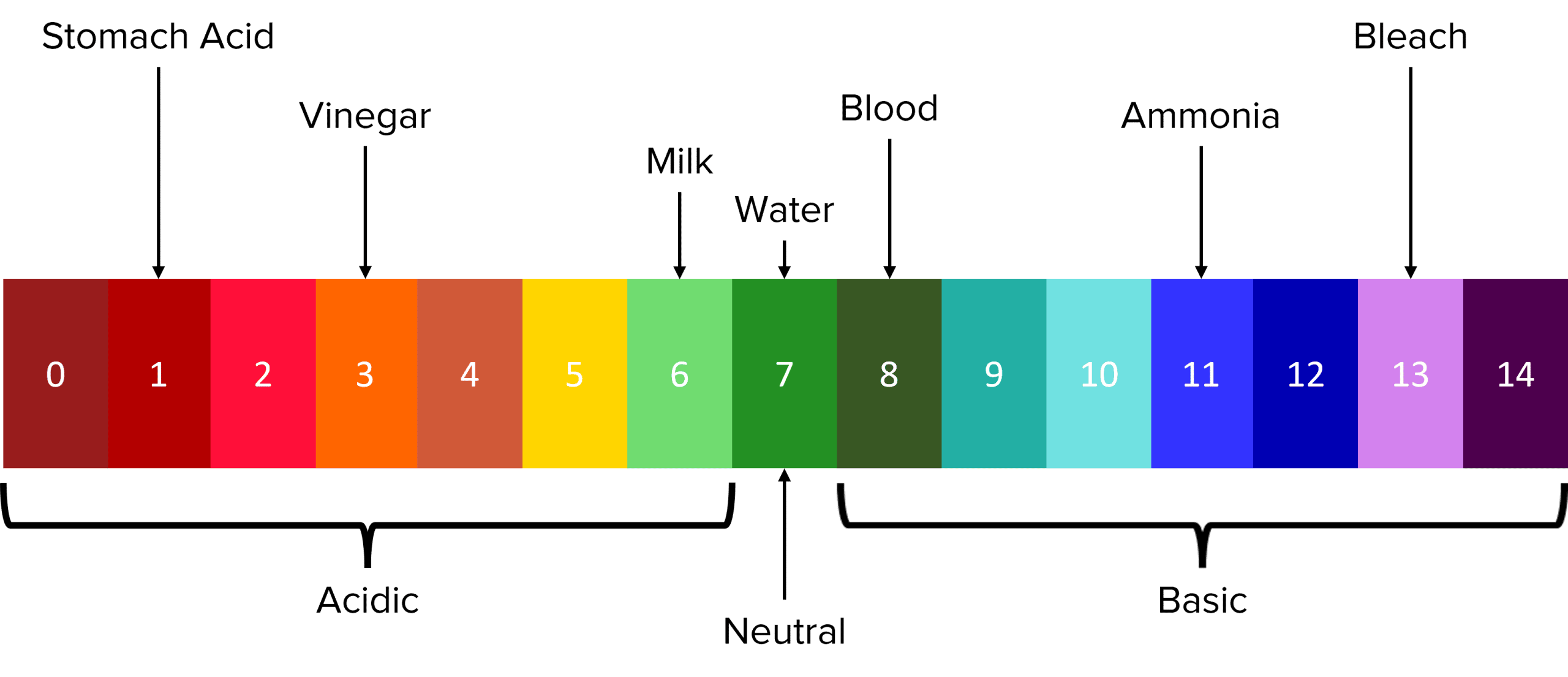The pH Scale is the most commonly used method to measure the acidity or basicity of a substance. The scale is a measure of the amount of $\text{H}^+$ ions that a substance will produce in solution (i.e. the concentration of $\text{H}^+$ ions, represented by $\left[\text{H}^{+}\right]$). The lower the value of its $\text{pH}$, the higher the concentration of $\text{H}^{+}$ ions.

The values of $\text{pH}$ range from $0$ to $14$ and have no units. Substances with a $\text{pH}$ of $7$ are said to be neutral, with acidity/ basicity increasing as the $\text{pH}$ moves further away from $7$.

Chemicals with $\text{pH}$ values that fall within the range $0$ to $6$ are know as acidic. This includes chemicals such as battery acid $(\text{pH } 0)$ and milk $(\text{pH } 6)$. As the $\text{pH}$ of a substance decreases, its acidity increases

Chemicals with $\text{pH}$ values that fall within the range $8$ to $14$ are know as basic (or alkaline). This includes chemicals such as ammonia $(\text{pH } 11)$ and blood $(\text{pH } 8)$. As the $\text{pH}$ of a substance increases, its basicity increases

The $\text{pH}$ of a substance can be measured using a universal indicator. Universal indicators are chemical mixtures that will react with substances across the whole pH range. When added to acids or bases (which are typically colourless), these reactions will produce solutions with colours corresponding to a specific $\text{pH}$ value in the scale. An example of the $\text{pH}$ colour scale is shown above.

GCSECombined Science FoundationCombined Science HigherChemistry FoundationChemistry HigherAQA

## Strong and Weak Acids

When dissolved in aqueous solutions, acids will dissociate. Dissociation is the process where by a chemical compound separates to form aqueous ions. When acids dissociate, they do so into $\text{H}^+$ ions and anions (e.g. $\text{Cl}-$ for $\text{HCl}$ or $\text{SO}_4^2-$ in $\text{H}_2\text{SO}_4$).

$\text{HX}_{\text{(s)}}\rarr\text{H}^+_{\text{(aq)}}+\text{X}^-_{\text{(aq)}}$

As acidity is governed by the concentration of $\text{H}^+$ ions in solution, the extent of dissociation will determine how strong or weak an acid is. Strong acids are those that completely dissociate in solution. This means that every molecule of the acid has separated out into its constituent ions. In these cases, the concentration of $\text{H}^+$ ions will be be high, leading to a low $\text{pH}$

In weak acids there is only partial dissociation. This means only a small proportion of the molecules in the solution will dissociate into solution. This dissociation is said to be reversible and so an equilibrium is set up:

$\text{HA}_{\text{(s)}}\rightleftharpoons\text{H}^+_{\text{(aq)}}+\text{A}^-_{\text{(aq)}}$

As only a small amount of the acid actually dissociates, the equilibrium is taken to lie far to the left. This means that $\left[\text{H}^+\right]$ will be much lower than in a solution of strong acid and so the $\text{pH}$ will be higher.

Strong acids are typically those made up of ionic compounds such as $\text{HCl}$, $\text{HNO}_3$, and $\text{H}_2\text{SO}_4$. Weak acids are typically those made from organic compounds such as ethanoic acid $\left(\text{CH}_2\text{COOH}\right)$.

As the concentration of $\text{H}^+$ ions, and therefore the strength of an acid, increases its $\text{pH}$ will decrease. The $\text{pH}$ will decrease by $1$ for every factor of $10$ increase in the concentration of $\text{H}^+$ ions. This can be expressed by the general formula:

$\text{Factor change in }\left[\text{H}^+\right]=10^{-X}$

where $X$ is the difference in $\text{pH}$

For example, if the $\text{pH}$ of a sample of acid is increased from $1$ to $3$ we can calculate the increase in $\left[\text{H}^{+}\right]$

\begin{aligned}\text{Factor change in }\left[\text{H}^+\right]&=10^{-X}\\ &=10^{-(1-3)}\\ &=10^{-(-2)}\\ &=100\end{aligned}

It is important to distinguish between acids that are strong and acids that are concentrated. A solution of a weak acid may have a relatively low $\text{pH}$ if it is highly concentrated as the concentration of $\text{H}^+$ ions will be relatively high. Similarly, a solution of a strong acid may have a relatively high $\text{pH}$ if its fairly dilute. In this case the $\text{H}^+$ concentration will be relatively low, despite the acid being a strong acid.

GCSECombined Science HigherChemistry HigherAQA

## Neutralisation Reactions

Acids and alkalis (bases) will react together in a process called neutralisation. Most neutralisations will follow the following general chemical equation:

$\text{Acid} + \text{Base} \rarr \text{Salt} + \text{Water}$

In neutralisation reactions, acids and and bases are mixed to form a solution or mixture that has a $\text{pH}$ of around $7$ (i.e. a neutral solution).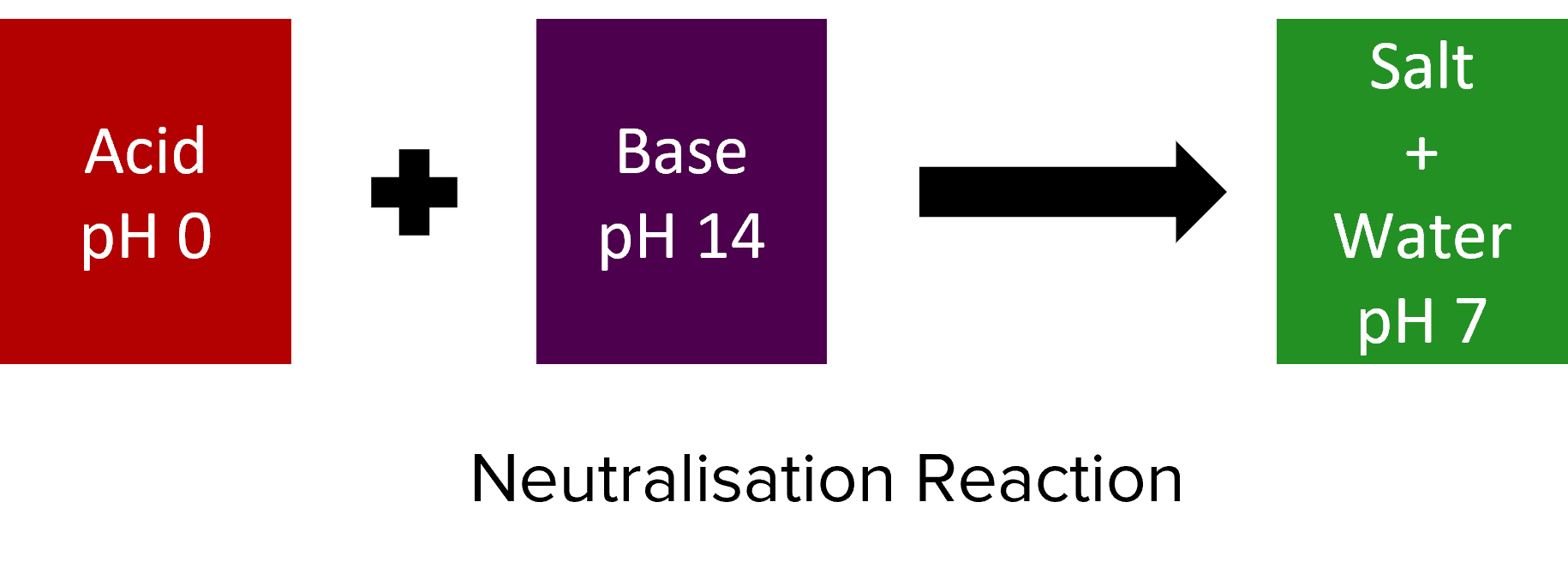Salts are chemical compounds – often solid – that form when acids and bases react. They consist of ionic compounds formed between the anions of the acid (i.e. the part of the acid that isn’t hydrogen) and the cations of the base (i.e. the metal element). The remaining $\text{H}^{+}$ ions of the acid and $\text{OH}^{-}$ ions of the base combine to form $\text{H}_2\text{O}$.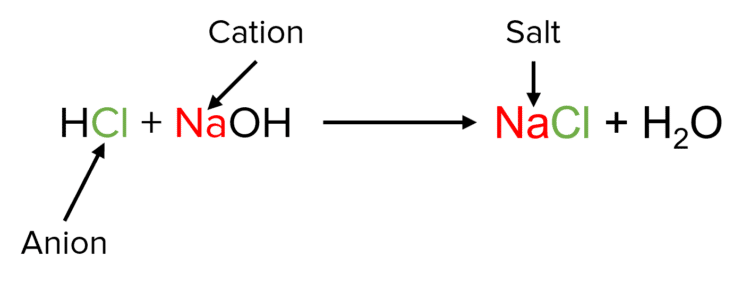Salts are typically insoluble in water. As a result of this, salts will often precipitate from a solution when they are formed. When a compound precipitates, tiny crystals are formed in solution. These crystals are more dense than the solvent and so fall to the bottom of the reaction vessel. The accumulation of these crystals at the bottom of the reaction vessel is called a precipitate. As salts are often insoluble, it is possible to separate them from solutions using filtration.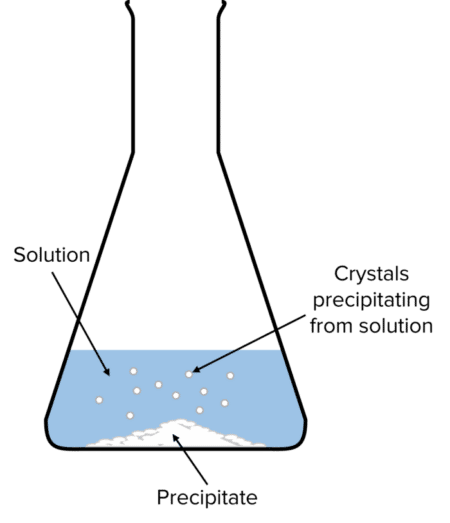GCSECombined Science FoundationCombined Science HigherChemistry FoundationChemistry HigherAQA

## Titrations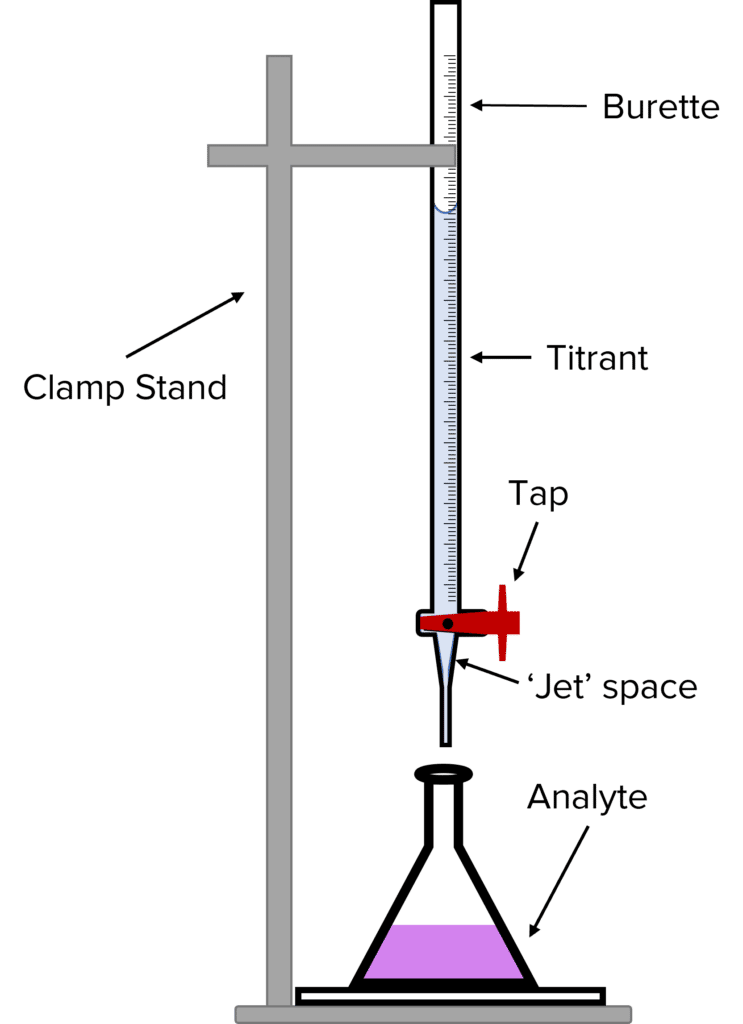Titrations are a type of experiment in chemistry that can be used to determine the volumes and concentrations of different chemicals required for a chemical reaction. The most common use of titrations it to find out how much of a strong base is needed to neutralise a strong acid or vise versa. This information can then be used to calculate the concentration of the acid/ base.

To perform a titration we first pour a known volume of the chemical we want to neutralise – known as the analyte – into a conical flask. We then add an indicator. The indicator will tell us when the analyte has been neutralised by changing colour. Next, we rinse a burette with the chemical that will be used to neutralise the analyte – this is known as the titrant.

Once the burette has been rinsed, we partially fill it with the titrant. The tap of the burette should be opened and some of the titrant allowed to run though. This ensure that the jet space of the burette is filled with titrant. We then fill the burette so that the meniscus (the U shape formed between the surface of the titrant and the glass walls of the burette) lines up with the $0$ mark of the burette.

To run the titration, we open the tap of the burette and allow the titrant to run into the analyte. When the indicator changes colour, we close the tap and read off the volume of titrant added, this volume is known as the titrant.

When performing titration experiments, it is important to get consistent results. For each titration, the volume of the titre should be calculated as the average of at least three concordant results (i.e. titres with volumes that are all within $0.20\text{ cm}^{-3}$ of each other).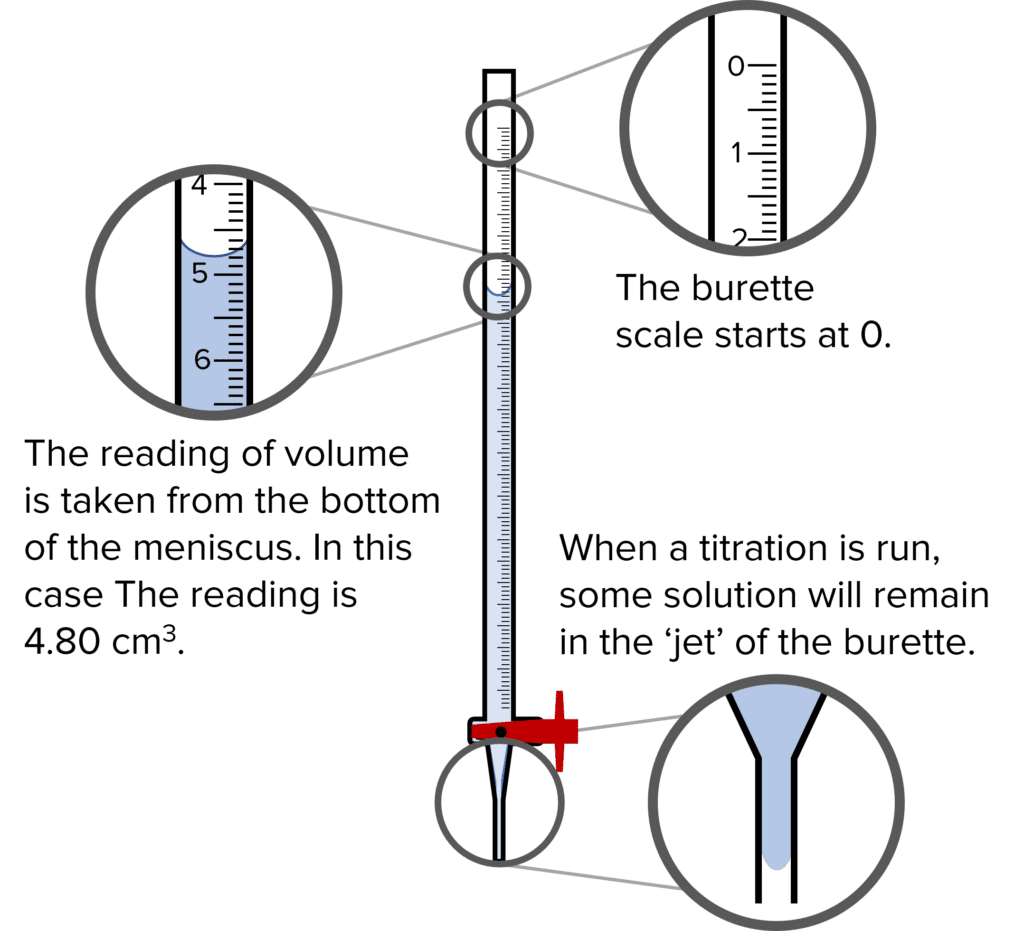The Burette

A burette is a specialized piece of equipment used to measure accurate volumes in chemistry. The narrow width of the burette creates a large meniscus in the solution it contains. This means that we can more accurately measure the volume of the solution. The narrow width also increases the resolution of the equipment, meaning that volumes can be read down to the nearest $0.05\text{ cm}^{-3}$

The tap of the burette also improves the accuracy of the volume reading. By changing the extent to which the tap is open, we can control the speed with which the titrant flows. By only opening the tap a little, we can add the titrant dropwise (one drop at a time). This allows us to add small and specific volumes with a high degree of control.

There are a few important things to bare in mind when using a burette.

1.  The volume scale of the burette begins at the top of the equipment at $0\text{ cm}^{-3}$. This is because the burette measures the volume that has been added to the analyte. As such when the burette is full, the volume reading is $0\text{ cm}^{-3}$.
2. When reading off the volume of a titrant, we measure from the bottom of the meniscus. If the bottom of the meniscus is between two of the scale lines then we take this as a reading of $0.05$. Volumes read from a burette should always be to two decimal places
3. When a burette is used, a small volume of the titrant will be contained in the jet space below the tap. Before running a titration, some titrant should be allowed to run through the burette to fill this space. If this is not done, the volume titre recorded will be too large. The extra volume is accounted for by the titrant held in the jet space.
GCSEChemistry FoundationChemistry HigherAQA

## Acid-Base Titrations

Method

1. Pour a $25\text{ cm}^{-3}$ sample of $0.1\text{ mol dm}^{-3}\text{ HCl}$ into a conical flask and add a few drops of phenolphthalein indicator. The solution in the flask should be colourless
2. Rinse a burette with a solution of $0.5\text{ mol dm}^{-3}\text{ NaOH}$. In doing so, ensure that the jet space is filled with the solution.
3. Fill the burette so that the meniscus of the $\text{NaOH}$ solution is in line with the $0$ reading of the burette.
4. Clamp the burette so that the jet feeds the $\text{NaOH}$ solution into the conical flask containing the acid and indicator. Place a white tile underneath the flask.
5. Open the tap of the burette so that a steady stream of $\text{NaOH}$ runs into the $\text{HCl}$ solution whilst swirling the flask.
6. When the solution in the flask turns pink the reaction is complete and the acid is neutralised. Close the tap and read off the volume of $\text{NaOH}$ added from the burette.
7. Repeat steps 1-6 until there are at least three concordant titres.
8. Repeat the experiment for different concentrations of $\text{HCl}$, keeping the concentration of $\text{NaOH}$ the same.

Analysis

Once a set of at least five average titrations has been collected, these should be plotted against the increasing concentration of the $\text{HCl}$ solution. As the concentration of the acid increases, we would expect that the volume of alkali needed to neutralise the acid will increase. This means that the average volume of the titre should increase as the concentration of acid increases. We would therefore plot of titre volume against acid concentration to look something like this: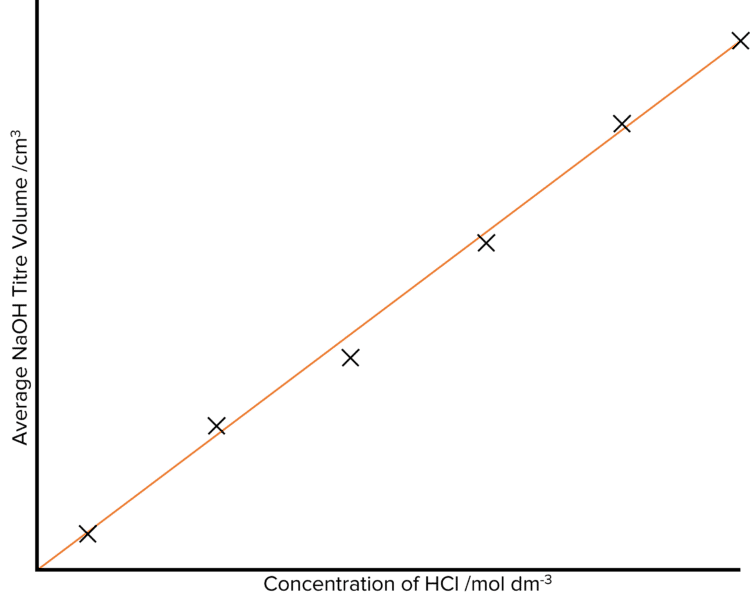GCSEChemistry FoundationChemistry HigherAQA

## Acids and Bases Example Questions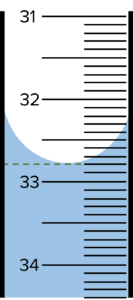$\text{Volume}=32.80\text{ cm}^{3}$Gold Standard Education

Acids are compounds that will form $\text{H}^+$ ions in aqueous solution.Gold Standard Education

$\text{pH }12$

(allow $\text{pH }11$)Gold Standard Education

Step 1: Calculate the change in $\text{pH}$.

\begin{aligned}\Delta\text{pH}&=3.5-1.6\\ &=-1.9\end{aligned}

Note, the change here is negative as the $\text{pH}$ is decreasing.

Step 2: Calculate the factor by which $\left[\text{H}^+\right]$.

\begin{aligned}\text{Factor change in }\left[\text{H}^+\right]&=10^{-\Delta\text{pH}}\\ &=10^{-(-1.9)}\\ &=79.43\end{aligned}Gold Standard Education

## Acids and Bases Worksheet and Example Questions

### Acids and pH

GCSEOfficial MMEProduct